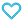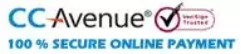# Problems in General Physics

130.00

Every chapter starts with important formulae of relevant areas of physics. The book comprises of around 1900 problems with hints for solving complicated problems. Physical constants and tables are also summarised at the end of the book.

829 in stock

Qty:Add to WishlistTable of Content:
• PART ONE : PHYSICAL FUNDAMENTALS OF MECHANICS
• 1.1 Kinematics
• 1.2 The Fundamental Equation of Dynamics
• 1.3 Laws of Conservation of Energy, Momentum and
• Angular  Momentum
• 1.4 Universal Gravitation
• 1.5 Dynamics of a Solid Body
• 1.6 Elastic Deformations of a Solid Body
• 1.7 Hydrodynamics
• 1.8 Relativistic  Mechanics
• Part Two : Thermodynamics  and Molecular  Physics
• 2.1 Equation of the Gas State. Processes
• 2.2 The First Law of Thermodynamics. Heat Capacity
• 2.3 Kinetic Theory of Gases. Boltzmann's Law and Maxwell's
• Distribution
• 2.4 The Second law of Thermodynamics Entropy
• 2.5 Liquids, Capillary Effects
• 2.6 Phase Transformations
• 2. 7 Transport Phenomena
• Part Three : Electrodynamics
• 3.1 Constant Electric Field in Vacuum
• 3.2 Conductors and Dielectrics in an Electric Field
• 3.3 Electric Capacitance. Energy of an Electric Field
• 3.4 Electric Current
• 3.5 Constant Magnetic Field. Magnetics
• 3.6 Electromagnetic Induction. Maxwell's Equations
• 3.7 Motion of Charged Particles in Electric and
• Magnetic Fields
• Part Four : Oscillations and Waves
• 4.1 Mechanical   Oscillations
• 4.2 Electric  Oscillations
• 4.3 Elastic Waves. Acoustics
• Part Five : Optics
• 5.1 Photometry and Geometrical Optics
• 5.2 Interference of Light
• 5.3 Diffraction of Light
• Problems in General Physics
• 5.4 Polarization of Light
• 5.5 Dispersion and Absorption of Light
• 5.6 Optics of  Moving  Sources
• 5.7 Thermal Radiation. Quantum Nature of Light
• Part Six: Atomic and Nuclear Physics
• 2.2 Scattering  of  Particles.  Rutherford-Bohr  Atom
• 2.3 Wave Properties of Particles. Schrodinger Equation
• 6.3 Properties of Atoms. Spectra
• 6.4 Molecules and Crystals
• 6.6 Nuclear  Reactions
• 6.7 Elementary Particles
• APPENDICES
• 1.  Basic Trigonometrical Formulae
• 2.  Sine Function Values
• 3.  Tangent Function Values
• 4.  Common Logarithms
• 5.  Exponential Functions
• 6.  Greek Alphabet
• 7.  Numerical Constants and Approximations
• 8.  SomeDataonVectors
• 9.  Derivatives and Integrals
• 10. Astronomical  Data
• 11. Density of Substances
• 12. Thermal Expansion Coefficients
• 13. Elastic Constants, Tensile Strength
• 14. Saturated Vapour Pressure
• 15. Gas Constants
• 16. Some Parameters of  Liquids  and Solids
• 17. Permittivities
• 18. Resistivities of Conductors
• 19. Magnetic Susceptibilities of Para and Diamagnetics
• 20. Refractive  Indices
• 21. Rotation of  the Plane of Polarization
• 22. Work Function of Various Metals
• 23. K Band  Absorption  Edge
• 24. Mass Absorption Coefficients
• 25. Ionization Potentials of Atoms
• 26. Mass of Light Atoms
• 27. Half-life Values of Radionuclides
• 28. Units of Physical Quantities
• 29. The Basic Formulas of Electrodynamics in the SI and
•       Gaussian  Systems
• 30. Fundamental Constants
ISBN11 9789385966590 I.E.IRODOV 2016 436 Class 11, Class 12 School Books Physics 368gm

## Reviews

There are no reviews yet.